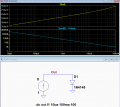# Problem with high and low of 555 astable timer

Joined Oct 25, 2020
12
I was looking at the circuit and made another attempt at it. What I did was use a multimeter to test the resistance offered by the diode. I then used the voltage-divider rule to solve for the voltage drop at the diode. Looking back at that I am not sure that was the right thing to do because the junction voltage was not included in the voltage drop. So now I might be much further away from fixing the problem. Any more suggestions?

#### Wolframore

Joined Jan 21, 2019
2,249
The diode is not a simple resistor, the drop for the exact same junction varies from current and temperature. Look up Shockley’s diode equation.

#### Tonyr1084

Joined Sep 24, 2015
5,855
Can you tell us what your desired time periods are?

#### crutschow

Joined Mar 14, 2008
26,968
What I did was use a multimeter to test the resistance offered by the diode.
That doesn't work to measure its "resistance" since a diode is not Ohmic, there is a logarithmic relation between the diode forward voltage and current [see typical small-diode simulation below that shows the forward voltage (yellow trace) and the pseudo spot resistance as calculated by Ohm's law (blue trace) versus diode current (horizontal axis)].

(Note: Don't confuse this spot resistance with the dynamic resistance of the diode, which is the AC resistance at its (arbitrary) operating point for a small AC signal, and is much smaller than this "spot resistance").

A multimeter will just give one spot on that curve, as determined by the amount of current it uses to measure resistance.
For example, if it used 1mA, then it would measure about 800 ohms of "resistance".
And since a multimeter changes the measurement current to change the resistance range, the diode "resistance" you measure depends upon the resistance scale you use.Last edited:

Joined Oct 25, 2020
12
The diode is not a simple resistor, the drop for the exact same junction varies from current and temperature. Look up Shockley’s diode equation.
I did. How do I determine the reverse saturation current though? If I could determine that I could use the silicon characteristics graph to get the voltage.

Joined Oct 25, 2020
12
Can you tell us what your desired time periods are?
I'm trying to get about 10 seconds high and 5 minutes low. The main problem is to understand the theory though. For instance, I would like to understand the correct equation for the high and low times.

#### Wolframore

Joined Jan 21, 2019
2,249
Keep in mind that the Size of the junction and the type of diode will also affect this ratio. Different diodes of the same part numbers will have slightly different curves. Why not just make it adjustable so you can fine tune it.

#### crutschow

Joined Mar 14, 2008
26,968
How do I determine the reverse saturation current though? If I could determine that I could use the silicon characteristics graph to get the voltage.
The diode forward drop doesn't vary that much between diodes.
My simulation graphs should work well enough for your purposes to get the forward drop at the diode current you have.

Joined Oct 25, 2020
12
The diode forward drop doesn't vary that much between diodes.
My simulation graphs should work well enough for your purposes to get the forward drop at the diode current you have.

#### crutschow

Joined Mar 14, 2008
26,968
Why can't you copy it from my post #24?

Or do your own graph using the free LTspice simulator program from Analog Devices.

•